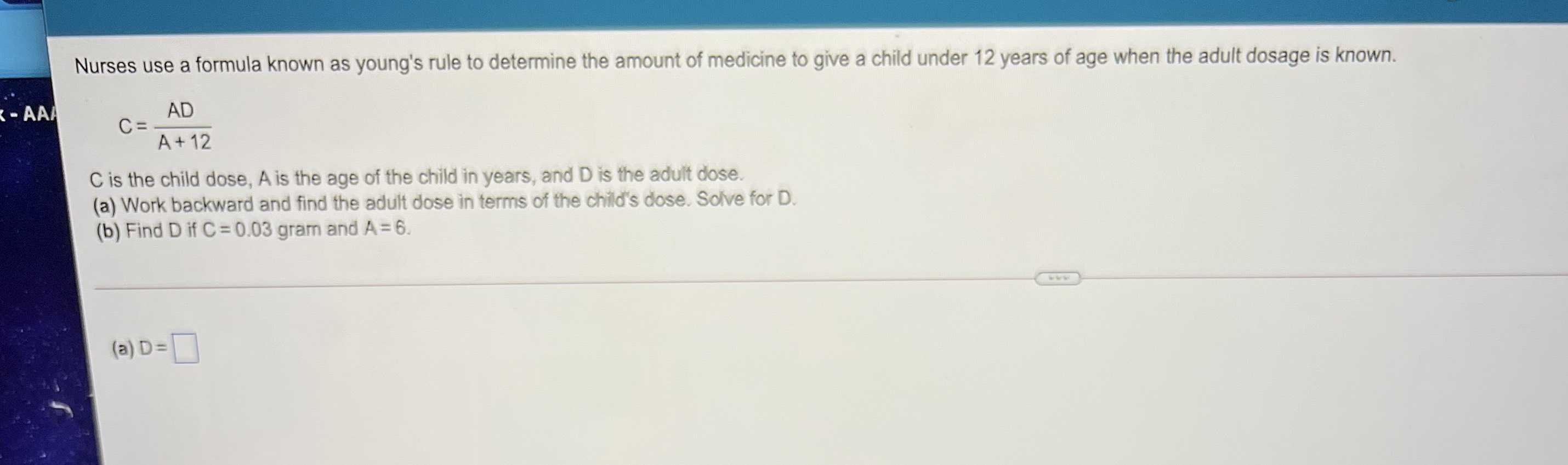### ¿Todavía tienes preguntas de matemáticas?

Pregunte a nuestros tutores expertos
Algebra
PreguntaA & B )

Nurses use a formula known as young's rule to determine the amount of medicine to give a child under $$12$$ years of age when the adult dosage is known.

$$C = \frac { A D } { A + 12 }$$

$$C$$ is the child dose, A is the age of the child in years, and $$D$$ is the adult dose.

(a) Work backward and find the adult dose in terms of the child's dose. Solve for $$D$$ .

(b) Find $$D$$ if $$C = 0.03$$ gram and $$A = 6$$ . (a) $$D =$$

$$\frac{C(A+ 12)}{A}$$